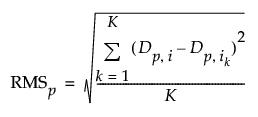Publication date: 05/24/2021

## K Nearest Neighbor Outliers

Use K Nearest Neighbor Outliers to identify an outlier based on distance to its nearest neighbor. For each value of k, the K Nearest Neighbor Outliers utility displays a plot of the Euclidean distance from each point to its kth nearest neighbor. You specify the largest value of k, denoted as K. Plots are provided for k = 1,2,3,5,...,K, using the Fibonacci sequence to avoid displaying too many plots.

Before the nearest neighbors are calculated, the columns are centered and scaled. The scaling factor is as follows:

max [Q(.75) - Q(.50), Q(.50) - Q(.25)] / [normalQuantile(0.75)]

where

Q(p) is the pth quantile

Note: If Q(75) or Q(25) are equal to the median, then more extreme quantiles are used until there is a non-zero range.

This approach is sensitive to the specified value of k. A small value of k can miss identifying points as outliers and a large value of k can falsely classify points as outliers:

Suppose that the specified K is small, so that you are studying only a few neighbors. If there is a cluster of more than K points that is far from the rest of the points, then the points within the cluster have small distances to their nearest neighbors. You might be unable to detect the cluster of outliers.

Suppose that the specified K is large, so that you are studying a large number of neighbors. If there are clusters with fewer than K data points, then the points within these clusters can appear to be outliers. You might overlook the fact that the points form a cluster, interpreting the individual cluster members as outliers instead.

### K Nearest Neighbor Outliers Report

When you select K Nearest Neighbor Outliers from the list of commands, you must specify the value of K to use as an upper bound for the farthest neighbor to be considered. You must also specify whether missing values should be imputed. Notice that K is set to 8 and Impute Missing is selected by default.

The report shows plots for select values of k up to the value K. The value of k for each plot is displayed in its vertical axis label. It is of the form Distance to Neighbor k = <a>, where a is an integer denoting the ath closest neighbor. Each plot shows the distance from the point in the ith row to its ath nearest neighbor. The points that have large distances from their neighbors, across multiple values of k, are likely to be outliers.

The buttons above the plots do the following:

Exclude Selected Rows

Excludes rows corresponding to selected points from further analysis. The rows are assigned the Excluded row state in the data table. You are asked if you want to rerun or close the K Nearest Neighbors report. Rerunning the analysis identifies new nearest neighbors. The plots are updated and the excluded points are not shown.

Scatterplot Matrix

Opens a separate window containing a scatterplot matrix for all columns in the analysis. You can explore potential outliers by selecting them in the K Nearest Neighbors plots and viewing them in the scatterplot matrix.

Save NN Distances

Saves the distances from each row to its nth nearest neighbor as new columns in the data table.

Close

Closes the K Nearest Neighbors report.

#### Largest Outliers

The report also includes a Largest Outliers table. This table contains the 20 observations with the largest distances from their Kth nearest neighbor. The table has the following columns:

Row

The row number of the observation.

Distance

The distance from the observation in the specified row and its Kth nearest neighbor. The table is sorted by this column in descending order.

Nearest Neighbors

Lists the row numbers for the k nearest neighbors. The first row number is the closest nearest neighbor. The last row number is the Kth nearest neighbor and the distance between this observation and the specified row is found in the Distance column.

Col<n>

Specifies the column name for the corresponding RMS value.

RMS<n>

Calculates the root mean squared differences across the k nearest neighbors for each column. The five largest RMS values are displayed in order, where RMS1 is the maximum RMS value. The pth RMS value is calculated as follows:where

Dp is the pth column

Dp,i is the value of the pth column for row i

Dp,ik is the value of the pth column for the kth nearest neighbor of row i

Note: The number of Col and RMS columns shown in the Largest Outliers table is the minimum of the number of columns specified in the launch and the number five.